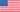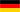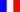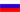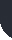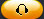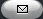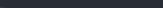Task Management Software for Team Collaboration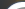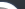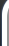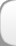HomeDownloadSupportPurchase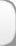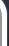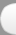Installation GuideStartUp GuideOnline Help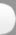# Total Rework Factor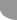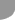Home  » Products  » VIP Task Manager Pro  » Tutorials  » Customization Guide  » Total Rework Factor

Total Rework Factor

Total Rework Factor is a key performance indicator that shows ratio of total cost of rework per phase against the total actual phase cost. It is presented by the formula below:

Total Rework Factor = Total Direct Cost of Rework per Phase / Actual Phase Cost

Total Direct Cost of Rework is actual total cost for work that is performed incorrectly and must be redone regardless of the initial reasons. Rework Cost belongs to separate items of operating costs.
Rework Cost includes cost for labor, materials and equipment.

General formula to calculate Direct Rework Cost looks like this:

Direct Rework Cost = (Rework * Standard Rate) + Resource per Use Costs + Task Fixed Cost

If project involves Direct Material, Direct Rework Cost is calculated by this formula:

Direct Rework Cost = (Rework * Standard Rate) + Resource per Use Costs + Actual Cost of Materials (per phase) + Other expenses

Other expenses may include cost for equipment, transportation, etc.

Actual Phase Cost is actual total cost spent per each project phase.

It is calculated using the formula as follows:

Actual Phase Cost = (Actual Phase Work * Standard Rate) + (Actual Phase Overtime Work * Overtime Rate) + Resource per Use Costs + Task Fixed Cost (actual per phase)

If Direct Material cost should be added Actual Phase Cost is calculated by the formula below:

Actual Phase Cost = (Actual Phase Work * Standard Rate) + (Actual Phase Overtime Work * Overtime Rate) + Resource per Use Costs + Actual Cost of Materials (per phase) + Other expenses (per phase)

Adding to the example about construction project, the given KPI may be used specifically to measure Total Field Rework Factor as a ratio of total Direct Cost of Filed Rework against Actual Construction Phase Cost.

Total Field Rework Factor = Direct Cost of Field Rework/Actual Construction Phase Cost

Along with Cost, Work, Schedule metrics Total Rework Factor KPI is usually used as additional measurement to get an overall picture of project performance.
Below are the instructions on how to measure Total Rework Factor:

• Add the custom field “Actual Phase Cost” with Code “cf_actual_phase_cost”, Type “Currency” and one of the abovementioned formulas.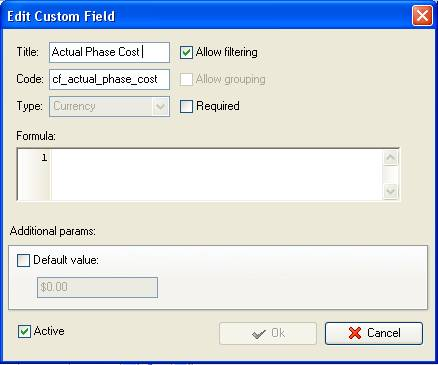You should take actual values per each project phase.

• Add the custom field “Direct Cost of Rework” with code “cf_direct_cost_of_rework” and Type “Currency”. Use one of the fomulas above to calculate Direct Cost of Rework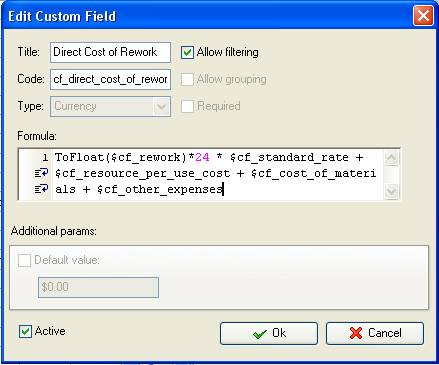• Add the custom field “Total Rework Factor” with Type “Float” and the formula:

(\$cf_direct_cost_of_rework/\$cf_actual_phase_cost) * 100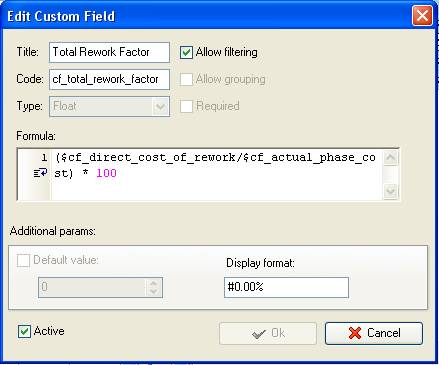• On Task Tree select the project phase for which you need to display Total Rework Factor and select “Custom fields options”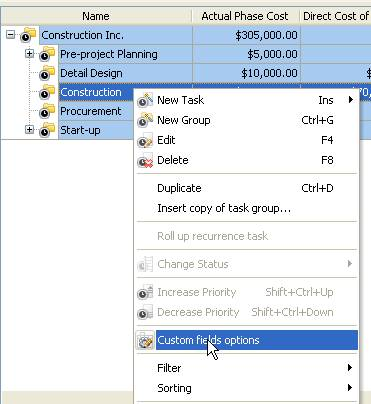• Set the following custom fields settings for the custom field “Actual Phase Cost” and “Direct Cost of Rework”

- In “Settings for” select “This task group”
- select “Enable”
- select “Sum”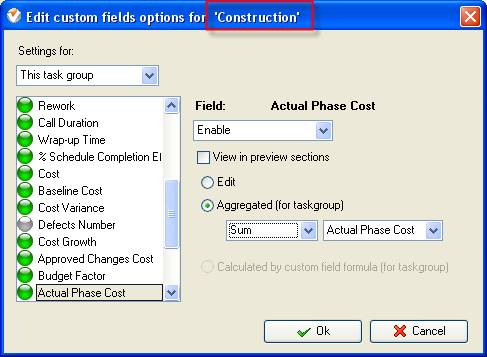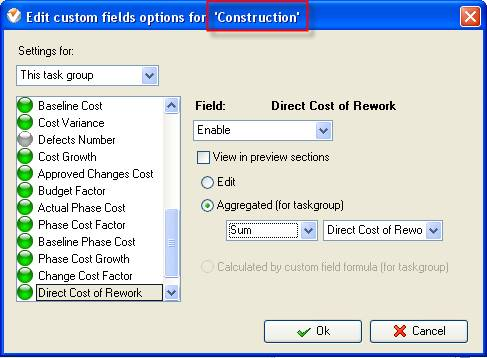• Set the following custom fields settings for the custom field “Total Rework Factor”

- In “Settings for” select “This task group”
- select “Enable”
- select “Calculated by custom field formula (for task group)”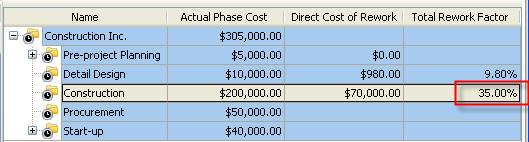Total Rework Factor on Task Tree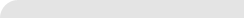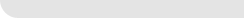» VIP Task Manager Pro VIP Task Manager Std Testimonials Turnkey Solution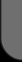ProductsSolutionsDownloadSupportPurchaseContactsForumVideoMy Account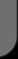Copyright © 2004 - 2023 Task Management Software